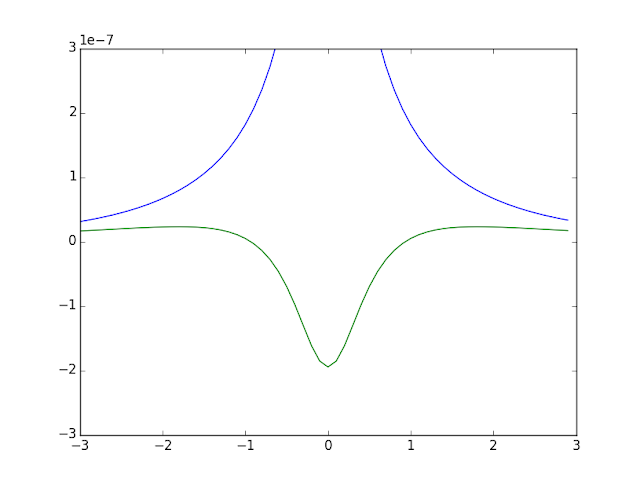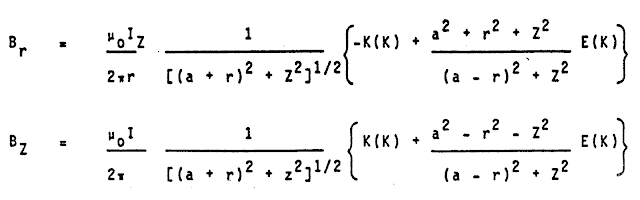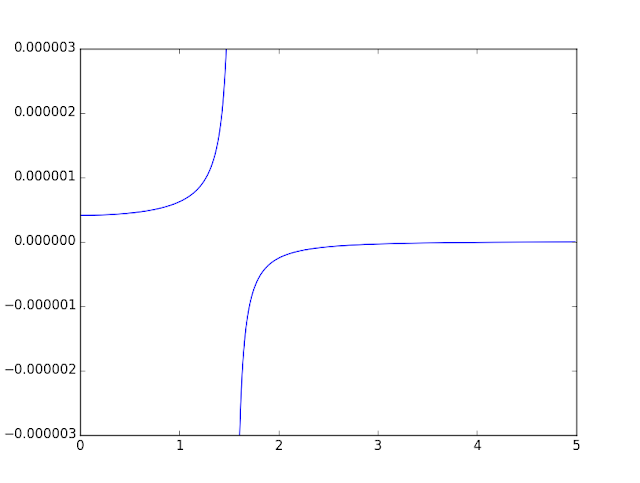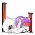## Wednesday, April 11, 2012

### I hope you find this blog enjoyable and helpful. Support it with a small donation.

Recently I was curious about the magnetic field generated by a circular current loop.  I had calculated the on axis field easily back in my MRI course at university, but we never went any further than that.  There is a good reason for that, it gets ugly, quickly.  Where you can solve the on axis case in about half a page, it take about 10 pages for the off axis case.

You would not believe how hard it was to find a worked solution to this on-line.  I never really did end up finding one, this is pieced together from textbooks, websites, and on-line lecture notes.  So I thought I would put them all together, inject a few of my ideas, and try to present a clear explanation of the solution.

Here is the PDF I used during the presentation and the wxMaxim file I used to calculate the final answer.

OffAxisMagneticField.pdf
OffAxisMagneticField.wxm

It was a good exercise really. I had to learn LaTeX, which I had wanted to do for a long time, and I found out about Maxima, a computer algebra system.  I used wxMaxima to solve the final part of the problem and was quite impressed.  It's layout and red blue interface made me feel like I was using Maple.  The range and quality of free software out there for Linux really amazes me, but I wouldn't mind my own copies of MATLAB, Maple, and Altium Designer.  While we're at it, throw in COMSOL Multiphysics too.

Update 3 August 2014

I've had a question about how you would calculate the circulating current in a thin disc.  My initial train of thought would be to split the disc up into infinitesimal rings calculate the magnetic field and integrate them, like this, but I feel there are some problems with this method.

Let me be clear, I don't actually know the answer, so I'll just list some of my concerns and ideas.  It's important to note that the current distribution in the disc may not be even.  The current circulating near edge has further to travel compared to current nearer the middle.  This may mean that there is less of it to give a constant voltage drop around the disc at all distances from the centre.

Another problem with this method is that you're calculating the magnetic field of each ring in isolation from the rest.  To calculate it properly you can consider each ring of current by itself, but you need to leave the conducting material of the disc behind as it will influence the shape of the magnetic field.  There could even be a problem with that as current from different rings may interact, so considering them in isolation may not be valid.

I'm sure someone could answer this problem, but at this point I'd reach for a finite element solver like www.femm.info (free) or www.comsol.com (paid, but beautiful)

Update 3 November 2015

Someone on YouTube asked a few questions about one of the equations in the video and at first I was worried I'd gotten it wrong, but everything's fine.  I'll post the question and then my response under it.  It's a good question and got me thinking about how the parts of the equation interact.

Question

Something seems wrong. On slide 12, for Bz(r,z). The term a^2-z^2-r^2 should probably be a^2-r^2. It is not physical that the field sign inverts for a certain z away from the loop. Moreover, assuming E2(0) = 1 and E1(0) = 0 (?), substituting r=0 will not result in the classical textbook equation of field at the center of a circular loop. Also, a factor π seems missing. (or E2(0) = π?)

Answer

The equation in question is quite complex and involves the interaction of elliptic integrals with other variables and is hard to tell what it should do, so I made a plot of the field for a coil with a radius of 1.55m with 1 Amp flowing through it.  The vertical axis is the z axis and the horizontal one is the radius.  The python code is here.  The arrows in the plot below only indicate direction, not magnitude of the field.Vector field of current loop
The question asks about the sign of the field in the z direction, and yes if you're inside the coil the component of the field in the z direction will always be positive (the blue plot at 1m radius). However, as soon as you move outside of the coil (the green plot at 2m radius) the direction of the field starts out positive, becomes negative, and swings back to positive.  So the field can switch direction if the radius of interest is greater than the coil radius.Magnitude of the magnetic field at r = 1 and 2 m along the z axis
The questioner almost answered the next part of the question themselves.  Setting r to 0 will make k zero.  The key is to then realise that E1(0) and E2(0) are both equal to pi/2.  From this point the equation reduces to the well know formula for the field on the axis of the coil.Reducing the off axis case to an on axis case

To improve confidence in my result I found an independent derivation of this equation in a patent from 1989 for MRI equipment.  The patent number is EP0310212 A2.corroborating independent equation

Update 28 November 2015

George asked in the comments about the in plane magnetic field of a current loop, i.e. z=0.  Due to symmetry Br will be equal to zero and there will only be a Bz component.  George supplied an equation that looks like it's on the right track (I haven't verified that but it has the structure that I'd expect).  My method of solving this would be to use the equation I've already calculated and set z to zero.

George also asks about when calculating the solution in Mathmatica it seems to hang.  I'm unfamiliar with Mathmatica, but I know the equation is undefined when r is equal to the radius of the current loop, so that could be an issue.

Also asked was how I calculated the values for the elliptic integrals.  When done numerically I use python with the package scipy.special.  To illustrate this process I've put together a small script that may help others get started.  It shows that in the plane of the coil the field starts out with a positive magnitude near the axis and rises as you approach the coil.  This is familiar behaviour of a solenoid, it has a reasonably uniform field near the centre of the coil.  Outside the coil the magnetic field is in the opposite direction but it tends to zero the further away from the coil you get.  Once again this makes sense.

I don't think I completely answered the question, but I hope it helps in some way.In plane magnetic field of coil with radius of 1.55 m and current of 1 Amp

Updated 4 December 2015

I've placed a worked solution for the in plane magnetic field of a circular current loop here

http://www.grant-trebbin.com/2015/12/in-plane-magnetic-field-of-current-loop.html

It starts with the Biot Savart law and progresses from there.

Update 13 April 2016

Where I've written E1(k) it should be E1(k^2) or E1(m) where m is k^2.  Same goes for E2.  I got a little mixed up with the notation.  There are a few inconsistencies in notation depending on what source you read.  The mathematics still work though.

### Support me and the blog with a small donation. I appreciate each one.

#### 36 comments:

1.David BillinghurstMay 13, 2012 at 10:38 PM

Nice. Maxima has the complete elliptic integral functions elliptic_kc and elliptic_ec. You can differentiate them and evaluate them for numerical args.

The graphics are nice. What package did you use?

1.Thanks for the tip. Maxima is full of surprises.

The graphics where done with Asymptote on the command line. I did a quick post about the process here

http://www.grant-trebbin.com/2012/02/ide-for-asymptote-using-inotifywait.html

The animation was done by creating a master Asymptote file then using a command line script to change the angle value in the file and render it to an image. The images were then combined into an mpeg for the animation. Very tedious.

I think there is a way to do it using TexMaker, but I haven't looked into that yet.

2.What would be needed if one wants to use Br and Bz you
obtained for a loop to calculate Br and Bz of a thin disk (or thin hollow disk, b=outer_radius - inner_radius) of a radius b?
( note: current goes parallel to a thin disk sheet in circular fashion,
like in (multiple) circular loop case: http://s8.postimg.org/xk8gszp05/disk_sheet.png )

Is just putting (I/b)*da instead of I to get dBz and dBr,
and then integrating them from a=0 to a=b (or a=inner_radius to a=outer_radius
in case of hollow disk) enough to get Bz and Br ?

--------------------------------------------------------------------------------------------------------------------

I've never heard of Maxima before. It's nice and free;)
Thank you, for letting me know about it.

1.Thanks for the interesting question.
That's a tough one and unfortunately I don't know the answer myself.
You've basically taking the same approach as I would, but the more I think about I don't know if it's right.
I've made a small update to the post with my thoughts and ideas.

Sorry I couldn't be more help. If you do find an answer let me know as I'm curious as well.

3.You are right about "The current circulating near edge has further to travel compared to current nearer the middle."

But looks like it can be neglected. Take a look at this:

1) http://www.ifi.unicamp.br/~assis/Phys-Rev-E-V68-046611(2003).pdf (page 9,where they discuss E_r , and get that Ef >> Er)

2) (annular disk. Does not take I=f(r) into account)
http://books.google.me/books?id=bDuOAQDk38gC&lpg=SA3-PA1&pg=SA3-PA9#v=onepage&q&f=false

(Would be nice to get a list of references from "Electromagnetic Shielding" book, and see how Haus calculates the H field !-;)

Will share when/if I have something more ;)

[little0nemo]

4.Hello! Great work. Most of it was over my head but I was looking for help on a homework problem that was similar to this but where z=0. I was told to approximate the B field off-axis by replacing the current loop with small dipole. Why was that not the approach taken here? Does the dipole method not work in some cases? Sorry for the broad question, I was just hoping for some conceptual clarification.

1.Thanks for the feedback. I have a few thoughts but I'm not entirely clear about your homework problem. Your use of the word dipole makes me think that you might be talking about a loop antenna? Usually this is for calculating far field radiation patterns, but the answers don't work nearby because of approximations used.

I suppose it's a similar process though, the integration I used treats the loop as a series of infinitesimally small current carrying wires.

I may be misunderstanding the problem you're tying to solve. If you could send a copy of the question I might be able to give a better answer. I'm curious if there's another way to solve it.

5.This comment has been removed by the author.

6.This comment has been removed by the author.

7.What is E1(k) and E2(k)?

8.I've found the formulas for every case : http://ntrs.nasa.gov/archive/nasa/casi.ntrs.nasa.gov/20010038494.pdf

1.Thanks for that link. It's a really good resource. E1(k) and E2(k) are elliptic integrals of the first and second kind.
It's not the standard notation of E(k) and K(k) but it all works out.

2.I've put a similar integral into the program mathematica and it just hangs. I want to calculate the B field at a point anywhere inside the plane of the current loop, the formula without using the magnetic potential I think is : http://i.imgur.com/tqLvtQI.jpg

3.By the way does the program you're using gives you the elliptic integrals or you have to calculate them yourself?

4.I've had a go at answering your question in an update to the main article. I've also added a small python script to show how I'd calculate the field in the plane of the current loop. I hope this can be helpful to you.

5.This comment has been removed by the author.

6.Thanks for the help Grant, I appreciate your answer, I should have clarified that the formula I gave http://i.imgur.com/tqLvtQI.jpg takes into account the speed of the electrons rather than the current of the loop with k=(μ0*q*v)/(8*Pi^2). I'm interested in a qualitative answer rather than a numerical approach , I'm trying to compare the force field F=Brωq that I got when using uniform rotating B fields in spherical coordinates to the answer from the biot-savart law but with the biot savart law I can't calculate the integrals properly to compare the answers, they should either cancel out or leave a term that I want to find out about.

7.Now I see what you're getting at. I just tried to work it out and got lost. One thing that might help is that you only need to calculate the field along the x or y axis. Due to rotational symmetry this is valid for all points the same distance from the loop axis. This means you can set x or y in your equation to 0. From what I can tell, there will still be elliptic integrals in the solution.

The problem is also discussed here.
https://www.physicsforums.com/threads/magnetic-field-in-plane-of-loop.232182/

I might have another crack at it tomorrow.

8.I've placed a worked solution for the in plane magnetic field of a circular current loop here

http://www.grant-trebbin.com/2015/12/in-plane-magnetic-field-of-current-loop.html

It starts with the Biot Savart law and progresses from there.

9.9.Is your k the standard k^2 = 4*r*a/(z^2 + (a + r)^2) and if so shouldn't the formula use EllipticE(k^2) and EllipticK(k^2)

1.Well spotted. It should be k^2 or m. Everything still works though. If I remember correctly the documentation for the software I was using got it wrong and I carried it through. It's a bit hard to change now, but I'll add a note to the post. Thanks for reading.

10.Your video is absolutely fantastic, and is the ONLY source on the internet I have found that finds the magnetic field off-axis using the magnetic vector potential--and with such ease. One question though. You find the vector potential first for all points in the x-z plane, one can generalise this for points in all of space by substituting $\phi'$ with $\phi'-\phi_1$, where $\phi_1$ is the angle that $\vec{OP}$ makes with the x-axis, can't one?

1.Thanks for the compliment. I'm not sure about that, I may not have understood your question correctly (I'm slightly confused about why you mention the angle between vec(OP) and the x-axis) but I don't think so. Working things out in the x-z plane makes things simple to calculate and then the radial symmetry generalises it for all of space. Technically can calculate the answer for any location of P, but it's easier to do it when it's in the x-z plane. It's been a while since I've read this so I'm very rusty. Sorry I couldn't give you a definitive answer.

2.Aah..I see now: one can simply rotate the coordinate system in order to generalise the solution. I get what you're saying about radial symmetry. Thanks.

11.This comment has been removed by the author.

12.you just made things really easy with your video, Thank you.

I'm really having a problem with calculating the field B for solenoid with core and I could not find any clear formula or code. Would you please help me with that?

1.I mean of the axis :)

2.I'm glad the video helped you out. I'd like to help but wouldn't know where to start. As soon as you start adding materials to the scenario it becomes complicated to the point that you really need to do numerical modelling.

3.Yes this is the case, that's why I'm struggling. Would you please recommend any reference?

Thank you

4.I feel like this is a problem that is beyond solving with equations. You also have the problem of hysteresis in the material. This makes the problem somewhat nonlinear. The only thing I can think of is trying some of the software in this list https://en.wikipedia.org/wiki/List_of_finite_element_software_packages I like COMSOL, but don't have access to it anymore. Another resource I can recommend searching is Google Books. There are some good physics textbooks in there. Some are restricted. some aren't. Sorry I couldn't be more help.

13.Hi Grant, thank you for your great work!
I have a similar script in Python for calculating the inductance of large coil (not a long solenoid). Now the thing that bothers me is that the B-field becomes essentially huge, if not infinite when the current filament is approached. The magnetic flux I get varies greatly depending on the step size in the script, since the closer it gets the current, the greater the flux gets. Now this doesn't happen in real world, flux and inductance is finite for a current loop. Obviously copper wire (and more so, 500 of them) is not infinitely thin filament.
I have found couple of calculators online for inductance, and they are in good agreement with my script (when using reasonable step size). These calculators seem to be using something else than Biot-Savart's law. I'm sure it is derived from Maxwell's equations, but I just can't find the derivation anywhere. My question is, if you could perhaps point me to the right direction how I could solve the problem with infinitely large magnetic field near the wire (in a coil), and also if you know how this approximation for inductance is derived: L ~ N^2*R µ_0 (ln(8R/a)-2), where R is radius of coil, a is radius of wire, N is number of loops and ~ should be "is approximately" For example here.

Only cases I can find are the calculations for magnetic forces in plasma streams in toroidal Tokamak reactors. Of course I can see a slight resemblance with my coil there ;).

This form of equation pops up for example in: This book and Wikipedia Magnetic pressure. I am still having hard time understanding where this all comes from.

Pete

1.First of all the problem with python sounds like something to do with numerical stability. It sounds like your equations are right but there is something wrong with the implementation.

That equation is strange. I've never seen it before but it pops up when you search for "short solenoid inductance" I'm finding references to something called Wheeler's formula from the early 1900's as well. I'll have another look tomorrow when I have some time.

14.Hello from Spain. First of all, thank you, Grant, for your video and work.

I was revisiting the typical problem of a conducting bar on conducting rails forming a complete loop with resistance R, in the presence of an external field B, and I noticed that in most books authors neglect the magnetic field created by the current around the loop itself. After doing some computatios, one can see that this magnetic field is neglegible compared to that of the external field after. But what's really remarkable is the fact that the magnetic field created by the current along the loop at any point of the loop itself is not zero. In the case of a circular loop, as is our case, the magnetic field at a point of the loop itself is not zero regardless what the plots may indicate. Why do you think this fact is so often ignored? Excuse my English if there were any mistakes.

1.Thanks for the compliment. It's an interesting question. I have to admit that I haven't come across this problem before and am not sure of the answer. I'd always thought the magnetic field from the induced current was an indirect statement of Lenz's law. From what I remember Lenz's law is usually stated as "The induced magnetic field inside any loop of wire always acts to keep the magnetic flux in the loop constant". I'm not sure that's right though. I might need to think about this some more.

2.I think I was a bit (actually, very) wrong in my first commment. I said: "the magnetic field created by the current along the loop at any point of the loop itself is not zero. In the case of a circular loop, as is our case, the magnetic field at a point of the loop itself is not zero regardless what the plots may indicate". Well, the plots, your plot 'In plane magnetic field of coil with radius of 1.55 m and current of 1 Amp' precisely says that the magnetic at an exact point of the loop itself (when r=1.55) is infinite. I did the calculations myself and the result I got was infinite, not zero like I said it was. I just didn't loot at the plots close enough!

This should not come as a surprise, since filiforms circuits are idealizations, like point charges are (this might be discussed). Real circuits have some volume and in that case I think the magnetic field along the axis of the circuit would probably be zero or approximately zero.

Lenz's law is usually stated like that, but as you suggest, that statement is a bit misleading, because the induced magnetic field never manages to keep the magnetic flux in the loop constant. The example I gave of a conducting bar on conducting rails is an example of that.